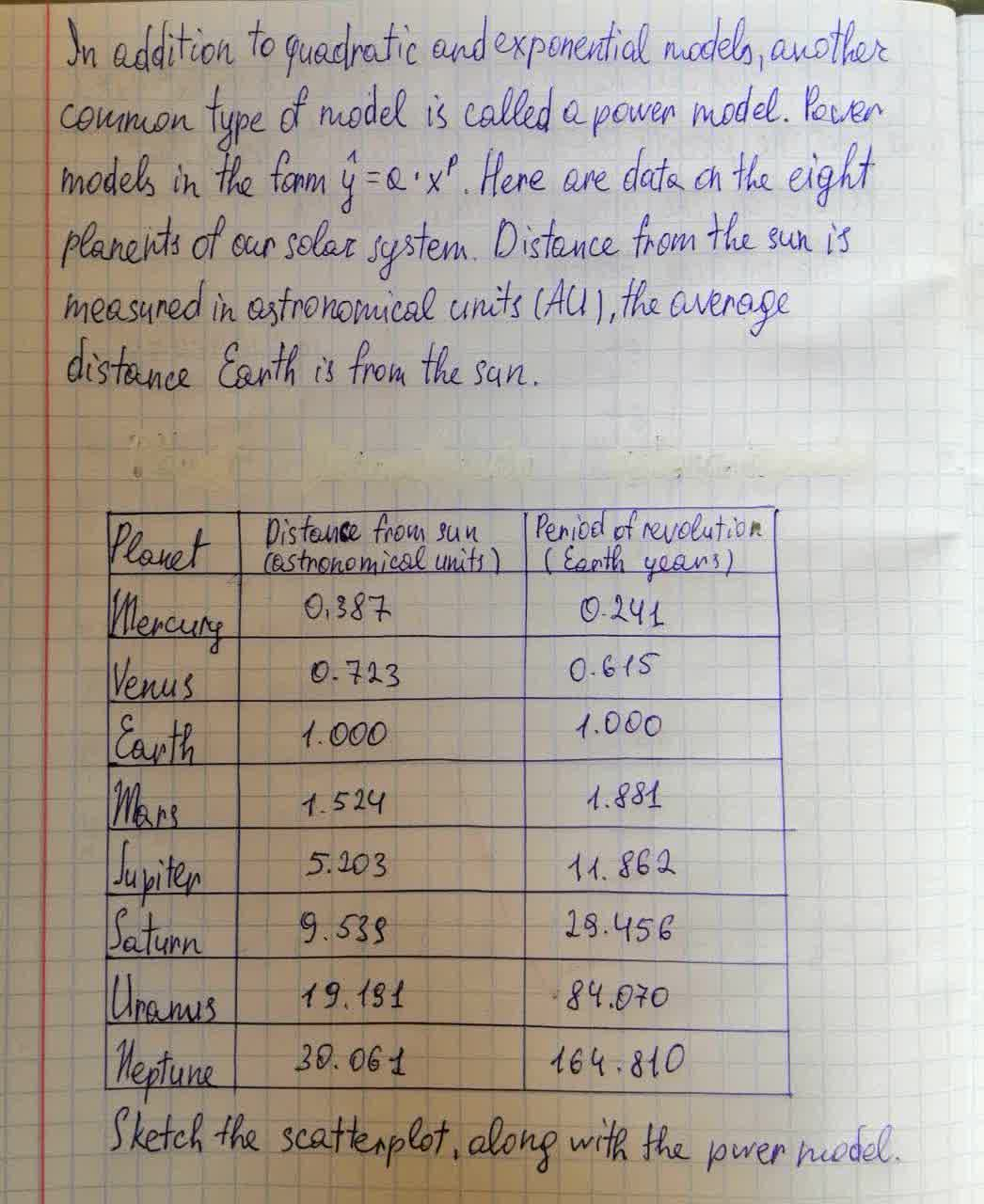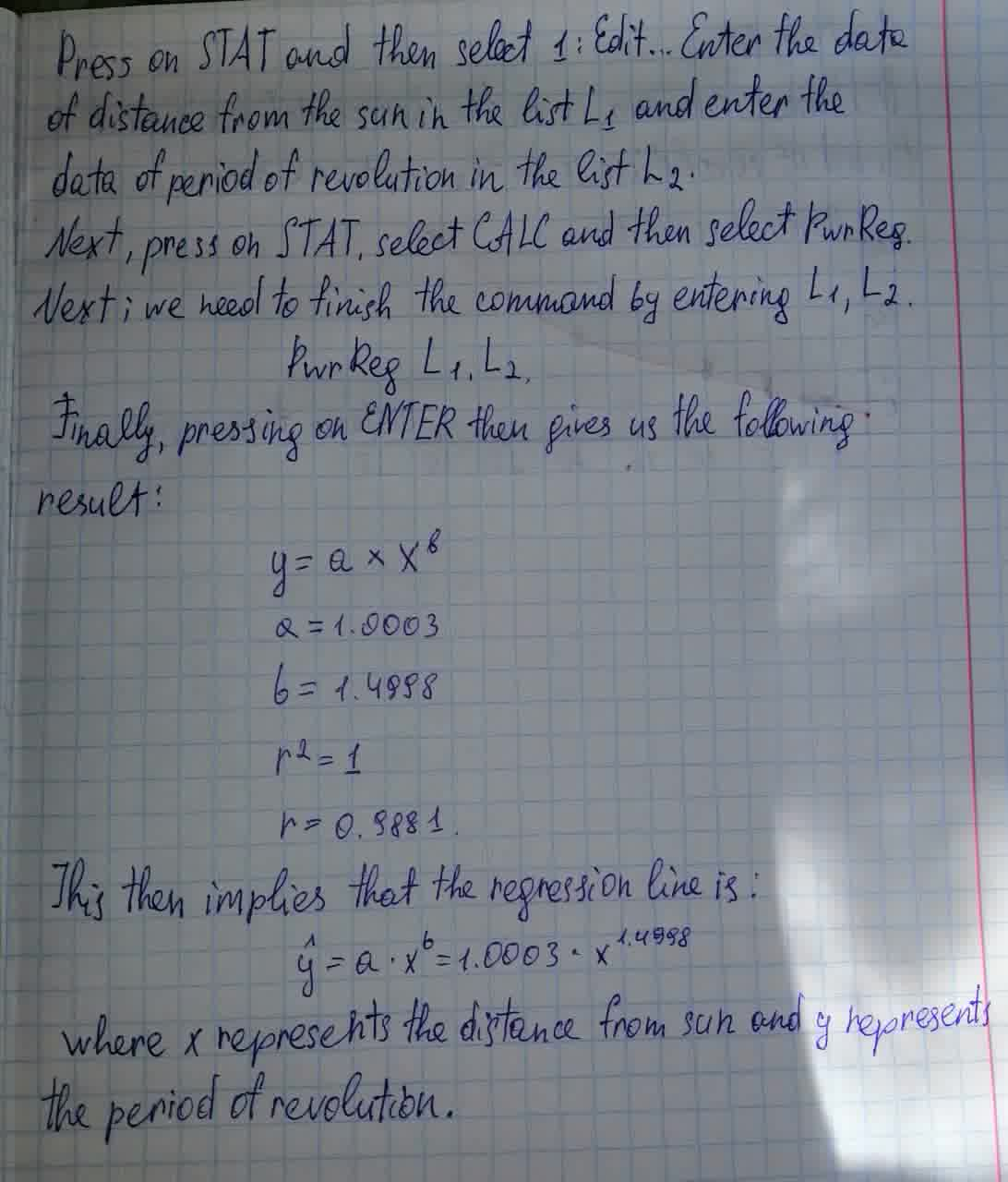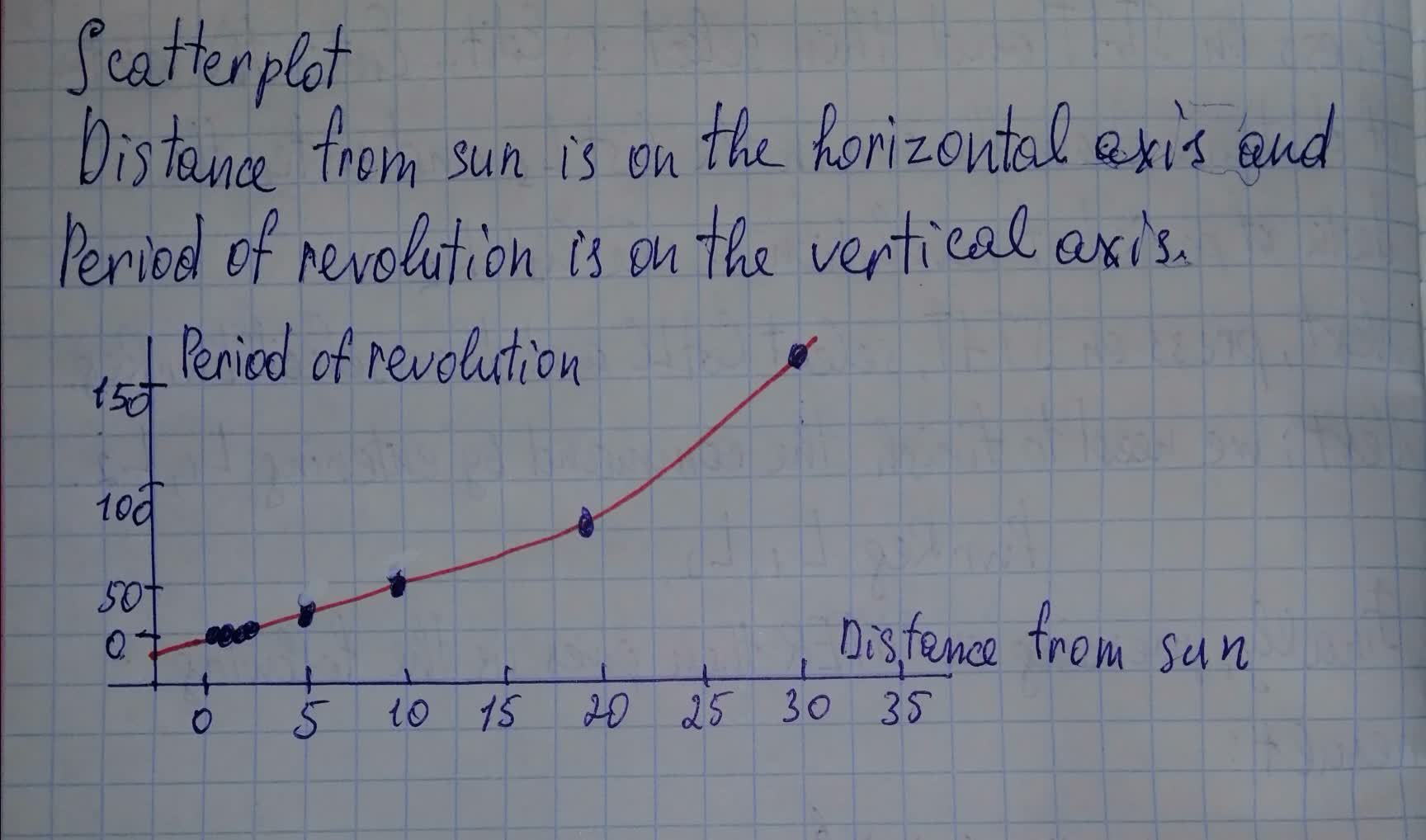# In addition to quadratic and exponential models, another common type of model is called a power model. Power models are models in the form \hat{y}=a \cdot x^{p}.ddaeeric 2021-07-20 Answered
In addition to quadratic and exponential models, another common type of model is called a power model. Power models are models in the form $\stackrel{^}{y}=a\cdot {x}^{p}.$ Here are data on the eight planets of our solar system. Distance from the sun is measured in astronomical units (AU), the average distance Earth is from the sun.You can still ask an expert for help

• Questions are typically answered in as fast as 30 minutes

Solve your problem for the price of one coffee

• Math expert for every subject
• Pay only if we can solve itgrbavit

We will carry out calculations to build a schedule